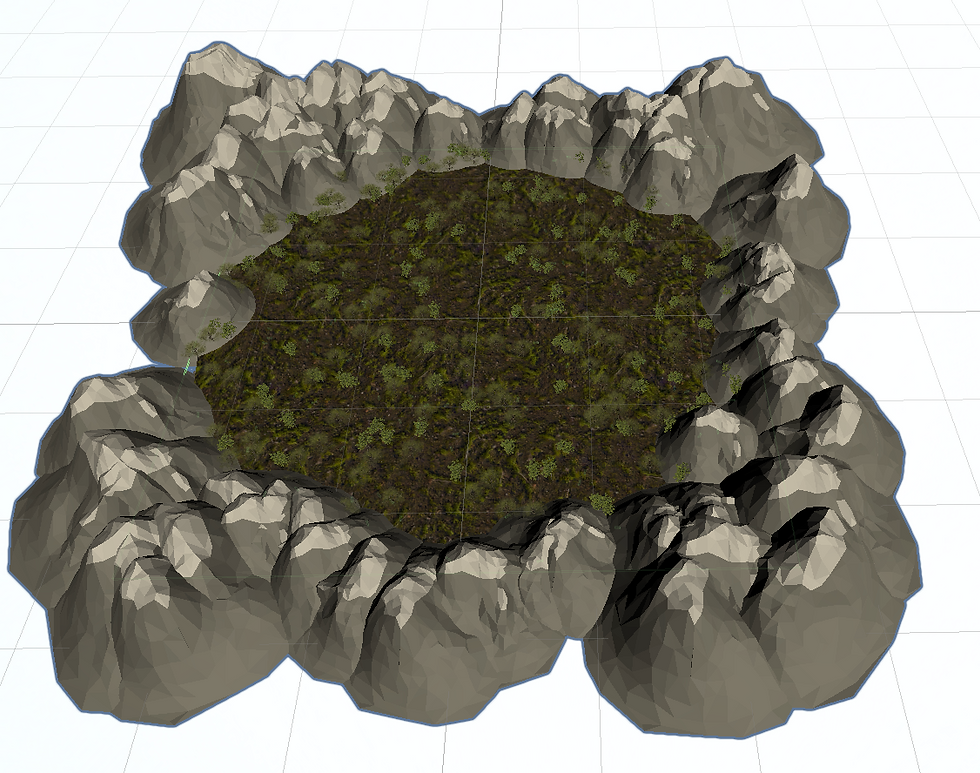top of page• Floney Yang

# Procedural Generation Algorithm

Overview

I created a procedural generation algorithm for generating a world with terrain, skybox, foreground/background/upground objects. This procedural generation algorithm focuses on generating several features of the world:

• All the foreground objects are randomly generated at any location in a circular flat terrain

• All the background objects are randomly generated in a “donut” shape range where the circular flat terrain will be placed at the centerProcess

1. Terrain Generation

I first created the flat terrain using Unity primitive object type cylinder and attached it with a box collider. I decided to use a simple geometry type because: 1) The background object can add bumps/heights to the overall terrain; 2) The material can make the flat terrain look realistic; 3) this method is simple and performance efficient compared with generating a semi flat surface using procedural generation that renders mesh triangles with different heights.2. Foreground/Background Objects Generation

Since we want objects to be randomly placed within the range we declared, the first step is to find the range for foreground/background objects. By drawing the world in a 2D top-down view, I noticed the foreground area and the background area can all be trapped in two squares while a circle will define a spherical range for objects to be within a certain range or without a certain range.

Foreground:Background:In the two graphs, we can see different colored sections can be represented using a certain x-axis range and z-axis range. So we can use one algorithm to define the range for both foreground and background object placement with only one variable being different: within/out of range. To solve this difference, I write a comparator to distinguish the range:

```bool Comparator(Vector3 randomSpawnPosition, float radius, bool withinRadius)
{
{
if (Vector3.Distance(randomSpawnPosition, transform.position) < radius) return true;
else return false;
}
else
{
if (Vector3.Distance(randomSpawnPosition, transform.position) > radius) return true;
else return false;
}
}```

After we define the coordinates of instantiation for each object, we want to find a way to balance the number of objects instantiated in each colored section, so that the smaller area doesn’t get overcrowded with objects while the larger area contains sparsely populated objects.

First we noticed the world is a symmetrical sphere. So I calculated the total area of the 4 colored blocks, and found the ratio between their area versus the total area. This ratio is used as an indicator for calculating how many objects should be spawn in each section based on a total object number the user declared. Since the shape is symmetrical, so left_num = right_num, up_num = down_num.

```float area = (minRange + maxRange) * 4 * (maxRange - minRange);
int left_num = (int)(2 * (maxRange - minRange) * maxRange / area * objectsNumber);
int up_num = (int)(2 * (maxRange - minRange) * minRange / area * objectsNumber);```I created four themes for this world's procedural generation. However, as the themes and background/foreground object numbers increase, there will be more and more time dedicated to drag the correct prefab under the correct public gameobject list. This method is inefficient because 1) memory efficiency: when the game only uses one theme, other theme’s objects are still being allocated in memory, 2) code style: code duplication in instantiation code to distinguish between theme, 3) time efficiency: this design is also inefficient for other designers who try to use the script and find it hard to drag models into the correct game object list.

To solve this problem, I used loading from the folder. All the needed assets are placed under a Resources folder where they are first separated by foreground/background/upground/terrain, then by themes. So designers can just drag assets into the correct folder without worrying about any content of the code. In this way, the memory remains relatively small with clean code style and less time used/confusion for external work.4. Final Results:

Reference

365 views
bottom of page